• 文本文件单词统计
2022-05-11 21:37:56
设计题目
1. 文本文件单词统计
1.1 【问题描述】
编写一个文本文件单词统计的程序，包括建立文件、单词统计、单词查询、单词定位的功能。
1.2 【基本要求】
程序应先询问用户的 ID (ID 号包括两个大写字母和 4 位数字 ) ，例如 :
请输入用户 ID : AB1234
程序应对输入的 ID 号验证，符合 ID 号要求的格式，然后程序提示四种选择 :
(1) 建立文件
(2) 单词统计
(3) 单词查询及定
(4) 退出
注意:
i) 文件至少包含 50 个英文单词（一定出现重复的单词，且一定包含数字）
ii) 文档不规范，单词之间可能不只有一个空格，并且有加引号的一些单词“ jiaozi
加引号的单词算单词，但数字不算单词
iii) 逐行扫描文本文件，计算文档中单词总数，及各个单词出现的频次，并且按照单词首字母 abcd ……
的顺序排列，显示并生成 soft.txt 文件
iv) 查询任意给出的单词是否存在，如果不存在，在屏幕上输出“ 查无此词！ ”；如果存在，显示单词
出现的总次数，并且详细列出其 出现的位置。
例如：
请您输入待查询的词： of
单词 of 共出现了 2 次；
1 次出现在第 1 行，第 5 个位置；
2 次出现在第 3 行，第 1 个位置。
请您输入待查询的词：

### 完整代码

#include<bits/stdc++.h>

using namespace std;

string s,word_text, words;
vector<string> words_single;   //存放要查找的单词 方便比较
vector<string> v;
vector<string> v3;
vector<string> v4;
int n,row,col,temp;
string j = "%";     //解决最后一次出现的位置为0的问题

int find_word(int col)
{
words_single.insert(words_single.end(),j);
for(int i=col;i<v3.size();i++)
{
words_single.insert(words_single.end(),v3[i]);
}

int sub_num2 = find(words_single.begin(),words_single.end(),words)-words_single.begin();
if(sub_num2<words_single.size())
{
n++;
col += sub_num2;
int temp_col = col;
if(col>11)
{

row = col/11+1;
temp_col = col%11;
}
printf("它在文件output中第%d次出现的位置位于第%d行第%d列\n",n,row,temp_col);
words_single.clear();
find_word(col);
}
else
{
return 0;
}
}

int main()
{
int flag=0;
bool sign = 0;
printf("请输入用户ID号：");
cin >> s;
vector<string> v2;
vector<int> word_num;
int num;  //去重后单词的个数
int sub_num;

//检查ID号格式是否正确   两个大写字母和四个数字
regex pattern("[A-Z][A-Z]\\d{4}");       //正则
sign = std::regex_match(s,pattern);
while(sign)
{
printf("请选择操作：\n");
cout << "1 建立文件" << endl << "2 单词统计" <<endl << "3 单词查询及定位" << endl <<"4 退出" <<endl;
scanf("%d",&flag);

string filename("output.txt");    //输入时文本的存储空间
fstream output_fstream;

string filename2("soft.txt") ;    //词频统计后单词的存储空间
fstream softfile;

if(flag ==1)  //建立文件
{
output_fstream.open(filename, std::ios_base::out);  //文件名 与打开方式
if (!output_fstream.is_open()) {
cerr << "Failed to open " << filename << '\n';
}
else {
cout << "请输入您的文本:" ;
cin.ignore();   //忽略换行 保证getline可以正确执行
getline(cin, word_text);

char e;
strcpy(e, word_text.c_str());   //将string转为char
const char *D = "^ ";  //遇到这些字符时不作处理
char *P;
P = strtok(e,D);

while(P)
{
v4.push_back(P);//在容器尾部加入一个数据
P=strtok(NULL,D);
}

for(int i=0;i<v4.size();i++)
{
if(i%11 == 0 and i!=0)
{
output_fstream <<  endl; //解决输入时 遇空格中断
}
output_fstream <<  v4[i] << " "; //解决输入时 遇空格中断
}

cerr << "输入成功，请打开'output.txt'文件自行查看" << endl<<endl;
}
output_fstream.close() ;
}

//对文档进行处理，使其规范化
char c;
strcpy(c, word_text.c_str());   //将string转为char
const char *d = "！\\" "@#￥%……&*（）—+=!$^&_}{}[];''""?></,\n 1234567890"; //遇到这些字符时不作处理 char *p; p = strtok(c,d); if(flag==2) //词频统计 { while(p) { v.push_back(p);//在容器尾部加入一个数据 p=strtok(NULL,d); } sort(v.begin(),v.end()); //先排序 后统计 for(int i=0;i<v.size();i++){ int cnt1 =0 ; //和计数方式有关 cnt1 = count(v2.begin(),v2.end(),v[i]); //如果这个单词之前没出现过 if(!cnt1) { int cnt = count(v.begin(),v.end(),v[i]); word_num.insert(word_num.end(),cnt); // 次数 v2.insert(v2.end(),v[i]); // 单词 } else { continue; } } //将结果写入到文件当中 softfile.open(filename2, std::ios_base::out); //文件名 与打开方式 if (!softfile.is_open()) { cerr << "Failed to open " << filename << '\n'; } else { for(int i =0;i<v2.size();i++) { cout << v2[i] << " " << word_num[i]<<endl; softfile << v2[i] << " " << word_num[i]<<endl; //解决输入时 遇空格中断 } cerr << "输入成功，请打开'soft.txt'文件自行查看" << endl<<endl; } output_fstream.close() ; } else if(flag==3) //单词查询及定位 { int col = 0; while(p) { v3.push_back(p);//在容器尾部加入一个数据 p=strtok(NULL,d); } n = 0; //置零，防止第几次叠加不是目标词的次数而出错 cout<< "请输入您要查询的词汇：" << endl; cin >> words; int cnt1 = count(v2.begin(),v2.end(), words); //判断目标单词是否存在 if(!cnt1) { cout << "查无此词"<<endl; } else if(cnt1) { cout <<"您输入的待查词是：" << words<<endl; sub_num = find(v2.begin(),v2.end(),words)-v2.begin(); //找到目标单词的下标 printf("单词出现的次数是：%d \n",word_num[sub_num]); //在存放次数的数组中找到次数 row = 1; find_word(col); } words.clear(); v3.clear() ; } else if(flag==4) { cout << "您已退出该程序"; break; } } while(!sign) { printf("ID号格式为两个大写字母和四个数字，SD形如：AB1234。 \n"); printf("ID号格式有误请重新输入 \n"); main(); } return 0; } ## 代码分析 ### 万能头文件 全局变量声明 #include<bits/stdc++.h> using namespace std; string s,word_text, words; vector<string> words_single; //存放要查找的单词 方便比较 vector<string> v; //用于存放初始输入经过规格化的文本 vector<string> v3; //工具vector vector<string> v4; //工具vector int n,row,col,temp; //n 第几次出现 row 行数 col 列数 temp工具人 string j = "%"; //解决最后一次出现的位置为0的问题  好的点是全局变量声明后，在整个程序段中都可以使用 然后，声明了四个vector数组 缺点就是占的空间比较大，优点的话是不会发生数据的错乱或者混淆 ### 功能三的实现 实现文件中单词的定位查询 int find_word(int col) //col 用于记录行数 { words_single.insert(words_single.end(),j); //j就是字符串"%" for(int i=col;i<v3.size();i++) { words_single.insert(words_single.end(),v3[i]); } //sub_num2 存储目标单词的位置 int sub_num2 = find(words_single.begin(),words_single.end(),words)-words_single.begin(); if(sub_num2<words_single.size()) { n++; col += sub_num2; int temp_col = col; if(col>11) { row = col/11+1; temp_col = col%11; } printf("它在文件output中第%d次出现的位置位于第%d行第%d列\n",n,row,temp_col); words_single.clear(); find_word(col); } else { return 0; } } 算法：切片，重新输入 然后定位查询 在words_single开始插入字符 “%”的原因是，占起址为0的空间，然后减的时候减去0刚好得到对应的位置，也就是单词对应的位置 col用作标志位，每次从col的下一个开始再次存入数组中，然后采用find对新的数组进行查找相关词，找到后，再次重找到位置的下一个切片存入数组中，再次遍历查询，直到没有找到目标词，然后退出 v3是在主函数中经过处理的 char 类型的数组，以一个单词为单位进行遍历 ### 主函数中依次实现四个功能 int main() { int flag=0; //标志用于指定进入哪个功能 bool sign = 0; // ID号是否符合格式的代码 printf("请输入用户ID号："); cin >> s; vector<string> v2; //字符型数组v2 vector<int> word_num; // int num; //去重后单词的个数 int sub_num; //功能2中查找对应单词出现次数的标志 //检查ID号格式是否正确 两个大写字母和四个数字 regex pattern("[A-Z][A-Z]\\d{4}"); //正则 判断是否符合格式要求 sign = std::regex_match(s,pattern); while(sign) //当符合要求后进入 { printf("请选择操作：\n"); cout << "1 建立文件" << endl << "2 单词统计" <<endl << "3 单词查询及定位" << endl <<"4 退出" <<endl; scanf("%d",&flag); //要进行哪一项操作 string filename("output.txt"); //输入时文本的存储空间 建立文件output fstream output_fstream; string filename2("soft.txt") ; //词频统计后单词的存储空间 fstream softfile; if(flag ==1) //建立文件 { output_fstream.open(filename, std::ios_base::out); //文件名 与打开方式 此处以out方式打开 if (!output_fstream.is_open()) { cerr << "Failed to open " << filename << '\n'; } else { cout << "请输入您的文本:" ; cin.ignore(); //忽略换行 保证getline可以正确执行 getline(cin, word_text); 将输入的内容全部接收 包括空格 char e; //空间大点，存的单词可以多 strcpy(e, word_text.c_str()); //将string转为char const char *D = "^ "; //遇到这些字符时不作处理 char *P; P = strtok(e,D); while(P) { //此处v4用于将文本以单词为单位输入 v4.push_back(P); //在容器尾部加入一个数据 P=strtok(NULL,D); } for(int i=0;i<v4.size();i++) { if(i%11 == 0 and i!=0) { output_fstream << endl; //解决输入时 遇空格中断 } output_fstream << v4[i] << " "; //解决输入时 空格消失 v4是一个char类型的没有空格的数组 } cerr << "输入成功，请打开'output.txt'文件自行查看" << endl<<endl; } output_fstream.close() ; //关闭文件 } //对文档进行处理，使其规范化 为功能二 词频统计做准备 char c; strcpy(c, word_text.c_str()); //将string转为char const char *d = "！\\" "@#￥%……&*（）—+=!$^&_}{}[];''""?></,\n 1234567890";  //遇到这些字符时，单词统计不作处理
char *p;
p = strtok(c,d);

if(flag==2) //词频统计
{
while(p)
{   //压入容器中便于遍历处理
v.push_back(p); //在容器尾部加入一个数据
p=strtok(NULL,d);
}

sort(v.begin(),v.end());    //先排序  后统计  按A-Z和a-z 的方式进行统计

for(int i=0;i<v.size();i++){
int cnt1 =0 ;                                //和计数方式有关
cnt1 = count(v2.begin(),v2.end(),v[i]);
if(!cnt1)                                    //如果这个单词之前没出现过
{
int cnt = count(v.begin(),v.end(),v[i]); //计数 统计该单词一共出现了几次
word_num.insert(word_num.end(),cnt);     //  word_num 容器 用于存放次数
v2.insert(v2.end(),v[i]);	             //  v2 用于存放单词
}   //需要注意的是，这个位置的单词和对应的次数 必须分别存放在数组下标相同的位置
else
{
continue; //如果这个单词出现过，就不再统计
}
}

//将结果写入到文件当中  该文件已 Key：value 方式存放
softfile.open(filename2, std::ios_base::out);  //文件名 与打开方式
if (!softfile.is_open()) {
cerr << "Failed to open " << filename << '\n';
}
else {
for(int i =0;i<v2.size();i++)
{
cout << v2[i] << " " << word_num[i]<<endl;     //在显示器上显示统计结果
softfile << v2[i] << " " << word_num[i]<<endl; //统计结果存入文档中，解决输入时 遇空格中断
}

cerr << "输入成功，请打开'soft.txt'文件自行查看" << endl<<endl;  //两次换行 界面简洁
}
output_fstream.close() ;   //文件关闭
}

else if(flag==3) //单词查询及定位
{
int col = 0;
while(p)
{
v3.push_back(p);//在容器尾部加入一个数据
p=strtok(NULL,d);
}
n = 0;     //置零，防止第几次叠加不是目标词的次数而出错
cout<< "请输入您要查询的词汇：" << endl;
cin >> words;
int cnt1 = count(v2.begin(),v2.end(), words);    //判断目标单词是否存在
if(!cnt1)
{
cout << "查无此词"<<endl;
}
else if(cnt1)
{
cout <<"您输入的待查词是：" << words<<endl;
sub_num = find(v2.begin(),v2.end(),words)-v2.begin();  //找到目标单词的下标
printf("单词出现的次数是：%d \n",word_num[sub_num]);          //在存放次数的数组中找到次数

row = 1;
find_word(col);  //调用查找功能
}
words.clear();
v3.clear() ;
}
else if(flag==4)
{
cout << "您已退出该程序";
break;
}
}

while(!sign)
{
printf("ID号格式为两个大写字母和四个数字，SD形如：AB1234。 \n");
printf("ID号格式有误请重新输入 \n");
main();
}
return 0;
}操作系统
更多相关内容
• 编写一个文本文件单词统计的程序，包括建立文件、单词统计、单词查询、单词定位的功能。 【基本要求】 程序应先询问用户的 ID号(ID 号包括两个大写字母和4 位数字)， 例如: 请输入用户 ID 号:AB1234 程序应对输入的 ...

# 【问题描述】

编写一个文本文件单词统计的程序，包括建立文件、单词统计、单词查询、单词定位的功能。

# 【基本要求】

程序应先询问用户的 ID号(ID 号包括两个大写字母和4 位数字)，
例如: 请输入用户 ID 号:AB1234 程序应对输入的 ID 号验证，符合 ID 号要求的格式，然后程序提示四种选择:

(1) 建立文件 (2) 单词统计 (3) 单词查询及定位 (4) 退出

注意:
i) 文件至少包含50个英文单词（一定出现重复的单词，且一定包含数字）
ii) 文档不规范，单词之间可能不只有一个空格，并且有加引号的一些单词“jiaozi” 加引号的单词算单词，但数字不算单词
iii) 逐行扫描文本文件，计算文档中单词总数，及各个单词出现的频次，并且按照单词首字母abcd…… 的顺序排列，显示并生成soft.txt文件
iv) 查询任意给出的单词是否存在，如果不存在，在屏幕上输出“查无此词！”；如果存在，显示单词 出现的总次数，并且详细列出其 出现的位置。

   例如：
请您输入待查询的词：of
单词 of 共出现了2次；
第1次出现在第1行，第5个位置；
第2次出现在第3行，第1个位置。


# 【具体代码】

public class total {
public static void main(String[] args) throws IOException {
Scanner scanf = new Scanner(System.in) ;
System.out.println("请输入用户的 ID 号");
String ID = scanf.next() ;
while(true) {
if(judge(ID)) {
System.out.println(""+ "(1) 建立文件\r\n" + "(2) 单词统计 \r\n" + "(3) 单词查询及定位\r\n"+"(4) 退出");
break;
}
else {
System.out.println("ID格式错误,请重新输入");
ID = scanf.next() ;
}
}

//不执行退出时始终为真
boolean rand = true ;
//选项
String choice;
List<String> list = new ArrayList<>();
while(rand) {
choice = scanf.next() ;
switch(choice) {
case "1": {
System.out.println("开始建立文件");
//每次读入一行

String a;
while(true){
if("-1".equals(a)) {
break;
} else {
}
}
createContentFile(list);
createSoftFile(list);
System.out.println("创建成功");
System.out.println(""+ "(1) 建立文件\r\n" + "(2) 单词统计 \r\n" + "(3) 单词查询及定位\r\n"+"(4) 退出");
break;
}

case "2":{
System.out.println("开始单词统计");
System.out.println(""+ "(1) 建立文件\r\n" + "(2) 单词统计 \r\n" + "(3) 单词查询及定位\r\n"+"(4) 退出");
break;
}
case "3":{
System.out.println("开始单词查询及定位") ;
System.out.println("请您输入待查询的词：");
String check = scanf.next();
while (!"-1".equals(check)){
checkWord(list, check);
System.out.println("请您输入待查询的词：  输入-1结束");
check = scanf.next();
}

System.out.println(""+ "(1) 建立文件\r\n" + "(2) 单词统计 \r\n" + "(3) 单词查询及定位\r\n"+"(4) 退出");
break;

}
case "4":{
System.out.println("成功退出") ;
rand = false;
break;

}

default: System.out.println(""+ "(1) 建立文件\r\n" + "(2) 单词统计 \r\n" + "(3) 单词查询及定位\r\n"+"(4) 退出");

}
}
}
/**判断id格式是否正确*/
public static boolean judge(String id) {

String regex = "^[A-Z]{2}[0-9]{4}$"; boolean flag = id.matches(regex); return flag; } /**创建ContentFile*/ public static void createContentFile(List<String> list) throws IOException { String filePath = "G:\\文本文件单词统计1\\生成文本"; File dir = new File(filePath); // 一、检查放置文件的文件夹路径是否存在，不存在则创建 if (!dir.exists()) { dir.mkdirs();// mkdirs创建多级目录 } File checkFile = new File(filePath + "/content.txt"); FileWriter writer = null; try { // 二、检查目标文件是否存在，不存在则创建 if (!checkFile.exists()) { checkFile.createNewFile();// 创建目标文件 } // 三、向目标文件中写入内容 // FileWriter(File file, boolean append)，append为true时为追加模式，false或缺省则为覆盖模式 //先清空文件 writer = new FileWriter(checkFile); writer.append(""); writer.flush(); //再追加写入 writer = new FileWriter(checkFile, true); for (String content : list){ writer.append(content); } writer.flush(); } catch (IOException e) { e.printStackTrace(); } finally { if (null != writer) { writer.close(); } } } /**创建SoftFile*/ public static void createSoftFile(List<String> list) throws IOException { //String为单词，countNum为单词出现的次数 Map<String, Integer> map = new HashMap<>(); int countNum = 0; //正则表达式 Pattern p = Pattern.compile("\\b[a-zA-Z-]+\\b"); Matcher m = p.matcher(list.toString()); //find方法扫描输入序列以查找与该模式匹配的下一个子序列 while(m.find()){ //String group()：返回匹配到的子字符串 String mstr = m.group(); //containsKey() 判断Map集合对象中是否包含指定的键名 if(map.containsKey(mstr)){ map.put(mstr, map.get(mstr)+1); }else{ map.put(mstr, 1); } countNum++; } Set<String> set = map.keySet(); String[] strings = set.toArray(new String[set.size()]); //忽略大小写排序 Arrays.sort(strings, String.CASE_INSENSITIVE_ORDER); String filePath = "G:\\文本文件单词统计1\\生成文本"; File dir = new File(filePath); // 一、检查放置文件的文件夹路径是否存在，不存在则创建 if (!dir.exists()) { dir.mkdirs();// mkdirs创建多级目录 } File checkFile = new File(filePath + "/soft.txt"); FileWriter writer = null; try { // 二、检查目标文件是否存在，不存在则创建 if (!checkFile.exists()) { checkFile.createNewFile();// 创建目标文件 } // 三、向目标文件中写入内容 // FileWriter(File file, boolean append)，append为true时为追加模式，false或缺省则为覆盖模式 writer = new FileWriter(checkFile, false); writer.append("共有单词:" + countNum + "个" + "\n"); for (String key : strings){ writer.append(key + ":" + map.get(key) + "次" + "\n"); } writer.flush(); } catch (IOException e) { e.printStackTrace(); } finally { if (null != writer) { writer.close(); } } } /**读取文件*/ public static void readFile() { FileReader fileReader = null; BufferedReader br = null; String line; try { // 判断文件是否存在 File testFile = new File("G:\\文本文件单词统计1\\生成文本\\soft.txt"); if(!testFile.exists()) { System.out.println(testFile.getName() + "文件尚未生成"); } // 读取文件 fileReader = new FileReader(testFile); br = new BufferedReader(fileReader); line = br.readLine(); while(line != null) { System.out.println(line); line = br.readLine(); } }catch(Exception e) { e.printStackTrace(); } finally { if(br != null) { try { br.close(); }catch(Exception e) { e.printStackTrace(); br = null; } } if(fileReader != null) { try { fileReader.close(); }catch(Exception e) { e.printStackTrace(); } } } } /**查询单词*/ public static void checkWord(List<String> list, String check){ Map<Integer,Integer> map = new HashMap<>(); int count = 0; for (int i = 0 ; i < list.size() ; i++){ int flag = list.get(i).indexOf(check); if (flag != -1){ map.put(i+1, flag+1); count++; } } if (count == 0){ System.out.println("查无此词！"); }else { System.out.println("单词" + check+"共出现了" + count + "次；"); int a = 1; for(Map.Entry<Integer, Integer> entry : map.entrySet()){ System.out.println("第" + a + "次" + "出现在第" + entry.getKey() + "行，" + "第" + entry.getValue() + "个位置"); a++; } } } }  # 【运行示例】 请输入用户的 ID 号 AD1234 (1) 建立文件 (2) 单词统计 (3) 单词查询及定位 (4) 退出 1 开始建立文件 what is the most important thing in the world? I think it is health You can take away our money，house，car，or even our clothes and we can survive. That is why we always try to eat in a healthy way and exercise regularlg. 0 -1 创建成功 (1) 建立文件 (2) 单词统计 (3) 单词查询及定位 (4) 退出 2 开始单词统计 共有单词:45个 a:1次 always:1次 and:2次 away:1次 can:2次 car:1次 clothes:1次 eat:1次 even:1次 exercise:1次 health:1次 healthy:1次 house:1次 I:1次 important:1次 in:2次 is:3次 it:1次 money:1次 most:1次 or:1次 our:2次 regularlg:1次 survive:1次 take:1次 That:1次 the:2次 thing:1次 think:1次 to:1次 try:1次 way:1次 we:2次 what:1次 why:1次 world:1次 You:1次 (1) 建立文件 (2) 单词统计 (3) 单词查询及定位 (4) 退出 3 开始单词查询及定位 请您输入待查询的词： we 单词we共出现了2次； 第1次出现在第3行，第63个位置 第2次出现在第4行，第13个位置 请您输入待查询的词： 输入-1结束 that 查无此词！ 请您输入待查询的词： 输入-1结束 That 单词That共出现了1次； 第1次出现在第4行，第1个位置 请您输入待查询的词： 输入-1结束 -1 (1) 建立文件 (2) 单词统计 (3) 单词查询及定位 (4) 退出 4 成功退出 Process finished with exit code 0  展开全文java • 文本文件单词统计（C语言） 本人目前还是一位在校大学生，写文章的目的是为了记录一下当前所学，与其他爱好者或从业者相互学习交流。文本文件单词统计这个题目是学校的一次课程设计中的一道，在编写时也是参考了很多... # 文本文件单词统计（C语言） 本人目前还是一位在校大学生，写文章的目的是为了记录一下当前所学，与其他爱好者或从业者相互学习交流。文本文件单词统计这个题目是学校的一次课程设计中的一道，在编写时也是参考了很多资料，借鉴了一些别人的想法，如有冒犯我会及时删除。同样，我的编写成果肯定会存在一些不足之处，也请读者多多包涵。 这个题目的具体信息如下： 1、问题描述 编写一个文本文件单词统计的程序，包括建立文件、单词统计、单词查询、单词定位的功能。 2、基本要求 程序应先询问用户的 ID号(ID 号包括两个大写字母和4 位数字)，例如:请输入用户 ID 号:AB1234 ，程序应对输入的 ID 号验证，符合 ID 号要求的格式（检验ID并不是主要要求，大家可自行忽略），然后程序提示四种选择: (1) 建立文件 (2) 单词统计 (3) 单词查询及定位 (4) 退出 注意: i) 文件至少包含50个英文单词（一定出现重复的单词，且一定包含数字） ii) 文档不规范，单词之间可能不只有一个空格，并且有加引号的一些单词“jiaozi” 加引号的单词算单词，但数字不算单词 iii) 逐行扫描文本文件，计算文档中单词总数，及各个单词出现的频次，并且按照单词首字母abcd…… 的顺序排列，显示并生成soft.txt文件 iv) 查询任意给出的单词是否存在，如果不存在，在屏幕上输出“查无此词！”；如果存在，显示单词出现的总次数，并且详细列出其出现的位置。 例如： 请您输入待查询的词：of 单词 of 共出现了2次； 第1次出现在第1行，第5个位置； 第2次出现在第3行，第1个位置。 请您输入待查询的词： 3、问题分析 解决如下问题： 1.检测用户ID格式是否正确，要求（1）用户ID位数为6；（2）前两位为大写英文字母，后四位是数字； 2.可创建文本文件并可输入内容； 3.对文本文件中的单词数进行统计，要求：（1）输出时将单词按照字典顺序排序；（2）数字不算入总数；（3）引号内的单词也算入总数； 4.实现单词定位，要求（1）输出单词出现的详细位置；（2）输出该单词出现的次数 1.4、功能实现 i.检验用户ID：用户从键盘中输入一个字符串。程序将接受的字符串赋值到一个字符数组，遍历数组中的元素判断格式是否正确。 ii.建立文本文件：(1）定义一个串变量;(2）定义文本文件;(3）输入文件名，打开该文件;(4）循环读入文本行，写入文本文件，其过程如下: While(不是文件输入结束){ 读入一文本行至串变量; 串变量写入文件; 输入是否结束输入标志;} (5）关闭文件。 iii.朴素匹配算法：该算法是整个单词检索程序的核心。对于主串的每一个字符作为子串的开头，与要匹配的字符串进行匹配，对主串做最大的循环，每个字符开头都做子串长度的小循环，直到匹配成功或者全部遍历完成为止。更具体地说：设有三个指针i,j,k,用i指示主串S每次开始比较的位置；指针j,k分别指示主串S和模式串T中当前正在等待比较的字符位置;一开始从主串S的第一个字符（i=0;j=1）和模式T的第一个字符(k=0）比较，若相等，则继续逐个比较后续字符(j++,k++)。否则从主串的下一个字符（i++）起再重新和模式串(j=0）的字符开始比较。依此类推，直到模式T中的所有字符都比较完，而且一直相等，则称匹配成功，并返回位置 i;否则返回-1，表示匹配失败。（可能讲述地不清楚，读者可再查询其他资料进行学习） iv. 单词统计： 单词总个数统计：在下文提到的单词出现频率统计的过程结束之后，将每个单词出现的次数相加就是单词的总个数。 单词之间有多个空格。在进行判断时，若空格之后仍然是空格，则多个空格作为一个空格进行处理。 单词出现频率统计：逐行扫描文本，将不同的单词放在一个字符数组中。在扫描过程中，每遇到一个与已保存在数组中的某一个单词相同的单词的时候，该单词的计数加1。 按字典顺序排序：将数组中的每个元素（即单词）采用冒泡法排序，最后输出详细的单词出现频率。 v.单词查询：遍历保存单词的字符数组，将要查询的单词与数组元素挨个比对。若不存在，则显示“查无此词”。 vi.单词定位：确定要检索的文本文件，确定要检索的单词，逐行扫描文本，将所要查询的单词与文本比对，若相同则立即输出行数和相应位置。 源代码如下： #include<stdio.h> #include<stdlib.h> #include<string.h> #include<time.h> typedef struct{ char str; //单词最大长度设为50 int cnt; //单词出现次数 }Str; //存放一个单词 int vcount(char* p){ //统计文本中单词个数 FILE *fp; char temp; //在进行冒泡排序时临时存放单词 char tmp; //用于在从文件中读取单词时临时存放单词 Str words; //单词数量上限(200) int num=0,count=0;//实际单词数量：num的值为不同的单词个数，count的值为总单词个数 int i,j,neww=1; //neww标志位，判断是否为新单词 int m,n; if((fp=fopen(p,"r"))==NULL){ printf("找不到文件\n"); return -1; } loop: while(fscanf(fp,"%s",tmp)!=EOF){ //fscanf从文件中挨个读取单个字符串 （单词） neww=1; for(i=0;i<50;i++){ //若读入的是数字则读取下一个字符串（因为数字不计入单词数） if(tmp[i]>='0'&&tmp[i]<='9'){ goto loop; } } for(i=0;i<50;i++){ //读入的字符串（单词）中可能包含标点符号",""."和自定义的结束标记"$" ，遇到这些符号将字符串断开
if(tmp[i]=='$'||tmp[i]==','||tmp[i]=='.') tmp[i]='\0'; } for(i=0; i<num; i++){ //重复的单词 if (strcmp(tmp, words[i].str)==0 ){ //和已经读取的单词进行比对，看是否为新单词。若不是，则单词出现次数加1 neww=0; words[i].cnt++; } } if (neww){ //若是新单词，则复制字符串进入word数组 for (j=0; tmp[j]!='\0'; j++) { words[num].str[j] = tmp[j]; } words[num].str[j] = '\0'; //单词末尾添加结束符 words[num++].cnt = 1; // 新单词数量+1 } } for(m=0;m<num-1;m++){ //采用冒泡排序实现单词的字典排序 for(n=0;n<num-1;n++){ if(strcmp(words[n].str,words[n+1].str)<0) //A>B,返回正数;A<B,返回负数;A=B,返回0 { strcpy(temp,words[n].str); //数字可以用“=”交换，字符串用strcpy函数 strcpy(words[n].str,words[n+1].str); strcpy(words[n+1].str,temp); } } } for(m=0;m<num;m++){ //统计单词总数 count += words[m].cnt; } printf("一共%d个不同的单词，每个单词出现次数如下：\n",num);//输出信息 for (i=num-1; i>=0; i--) { printf("%-10s %2d\n", words[i].str, words[i].cnt); } printf("单词个数为(包含重复出现)%d\n",count); fclose(fp); } int Index(char* p){ //朴素模式匹配算法 int j=0,i=0;//j用于记录输入的子串的长度,i用于记录主串的长度 char S,T; //s[]存放文件中的字符，t[]存放要搜索的单词 char ch1,ch2; FILE *fp; if((fp=fopen(p,"r"))==NULL){ printf("找不到文件\n"); return -1; } ch1=fgetc(fp); //将文件读取到一维数组当中 while(ch1!='$'){
S[i]=ch1;
i++;
ch1=fgetc(fp);
}
S[i]='$'; printf("请输入你要搜索的单词:\n"); //建立子串（输入想要搜索的单词） scanf("%s",T); ch2=T; while(ch2!='\0'){ //j代表了所要搜索的单词的长度 j++; ch2=T[j]; } //朴素模式匹配算法实现 int row=1,col=1;//row为行，col为列 int m=0,n=0,q=1;//m为S（主串）的下标，n为T（子串）的下标，q用于换行时记录m的值 int num=0;//num用于记录选定单词出现的次数 int left=0;//判断左右引号 while(m<i){ if(S[m]=='"'){ //加引号的单词处理：跳过引号，读取下一个字符，并设置标记left=1 m=m+1; left=1; } if(S[m]==T[n]){ m++; n++; } else{ m=m-n+1; n=0; }; if(n==j&&S[m+1]!=' '){ //匹配到相应单词 num++; //出现次数加1 if(row==1){ col=m-n+1; printf("第%d次出现的位置位于第%d行第%d列\n",num,row,col); } else{ col=m-n-q; printf("第%d次出现的位置位于第%d行第%d列\n",num,row,col); } } if(S[m]==' '||(S[m]=='"' &&left==1)){ //遇到右引号的情况下和空格进行一样的处理 col++; left=0; while(S[m]==' '||S[m]=='"') m=m+1; } if(S[m]=='\n'){ row++; q=m; }; if(S[m]=='$'){
printf("所选单词出现的次数是%d\n",num);
return num;
}
}
}

void createfile(char* p){									//创建文件
FILE *fp;
char ch;
fp=fopen(p,"w+");
ch=getchar();											//写入文件
printf("请输入单词:（请以$符号结束）\n"); while(ch!='$')
{
ch=getchar();
fputc(ch,fp);
}
fclose(fp);
}

int checkID(char s[],int x) {                              // 检验ID  ID格式；共6位 前两位大写字母 后四位为数字
int i = 0,y = x;
char t;
if(strlen(s)!=6){
printf("您输入的格式不正确（位数不是6位），请重新输入!\n");
return 1;
}
for(i=0;i<y;i++){
t[i] = s[i];
}
for(i=0;i<y;i++){      //判断前两位
if(i<2){
if(t[i]>='A'&&t[i]<='Z')  ;
else {
printf("您输入的格式不正确（前两位不是大写英文字母），请重新输入!\n");
return 1;
break;
}
}
if(i>=2){          //判断后四位
if(t[i]>='0'&&t[i]<='9')   ;
else {
printf("您输入的格式不正确（后四位中包含非数字字符），请重新输入！\n",i+1);
return 1;
break;
}
}
}
}

int main(){
int j = 1,l = 0;
int count=-1,c=-1;
char filename,afilename;
char *p;
char ID;
char quit;
while(j==1){                   // 调用cheakID函数对用户名进行判断
printf("请输入您的ID!（前两位为大写字母，后四位为整数）\n");
scanf("%s",ID);
j = checkID(ID,sizeof(ID));
if(j==0){
printf("输入正确！");
}
}
while(true){
printf("请选择您想要进行的操作（请输入操作前对应的数字）\n  1.建立文件\n  2.单词查询及定位\n  3.单词统计\n  4.退出\n");
scanf("%d",&l);
fflush(stdin);			//吃掉回车符
if(l!=1&&l!=2&&l!=3&&l!=4){
printf("您的输入有误，请重新输入！");
}
if(l==1){
printf("请输入所要创建的文件名（包括扩展名）");
scanf("%[^\n]",filename);
p=filename;
createfile(p);
printf("文件创建成功！");           // 建立文件
}
if(l==2){           					// 具体单词定位及查询
while(count==-1){
printf("请输入文件名（包括扩展名）");
scanf("%[^\n]",afilename);
fflush(stdin);
p=afilename;
count=Index(p);
if(count==0){
printf("查无此词！");
}
if(count==-1){
printf("文件名有误，请重新进入查询！\n");
break;
}
}
}
if(l==3){
while(c==-1){
printf("请输入文件名（包括扩展名）");
scanf("%[^\n]",afilename);
fflush(stdin);
p=afilename;
c=vcount(p);
if(c==-1){
printf("文件名有误，请重新进入查询！\n");
break;
}
}
}
if(l==4){
printf("您已退出该程序！");
break;                    			//退出练习程序
}
}
}


部分运行结果截图：展开全文c语言 数据结构 字符串 算法
• 文本文件单词统计 1.1 【问题描述】 编写一个文本文件单词统计的程序，包括建立文件、单词统计、单词查询、单词定位的功能。 1.2 【基本要求】 程序应先询问用户的ID号(ID 号包括两个大写字母和4 位数字)，例如: 请...

### 文章目录

文本文件单词统计
1.1 【问题描述】
编写一个文本文件单词统计的程序，包括建立文件、单词统计、单词查询、单词定位的功能。
1.2 【基本要求】
程序应先询问用户的ID号(ID 号包括两个大写字母和4 位数字)，例如:
请输入用户ID号:AB1234
程序应对输入的ID 号验证，符合ID 号要求的格式，然后程序提示四种选择:
(1) 建立文件
(2) 单词统计
(3) 单词查询及定位
(4) 退出
注意:
i) 文件至少包含50个英文单词（一定出现重复的单词，且一定包含数字）
ii) 文档不规范，单词之间可能不只有一个空格，并且有加引号的一些单词“jiaozi”
加引号的单词算单词，但数字不算单词
iii) 逐行扫描文本文件，计算文档中单词总数，及各个单词出现的频次，并且按照单词首字母abcd……
的顺序排列，显示并生成soft.txt文件
iv) 查询任意给出的单词是否存在，如果不存在，在屏幕上输出“查无此词！ ”；如果存在，显示单词
出现的总次数，并且详细列出其出现的位置。
例如： 请您输入待查询的词： of
单词of 共出现了2次；
第1次出现在第1行，第5个位置；
第2次出现在第3行，第1个位置。
请您输入待查询的词：

读完题后，我们可以把这个题目分成五个小模块，每个模块都可以用一个函数来实现，题目要求的单词查询和单词定位在一个函数内，但是我们会分开讲解这两部分功能。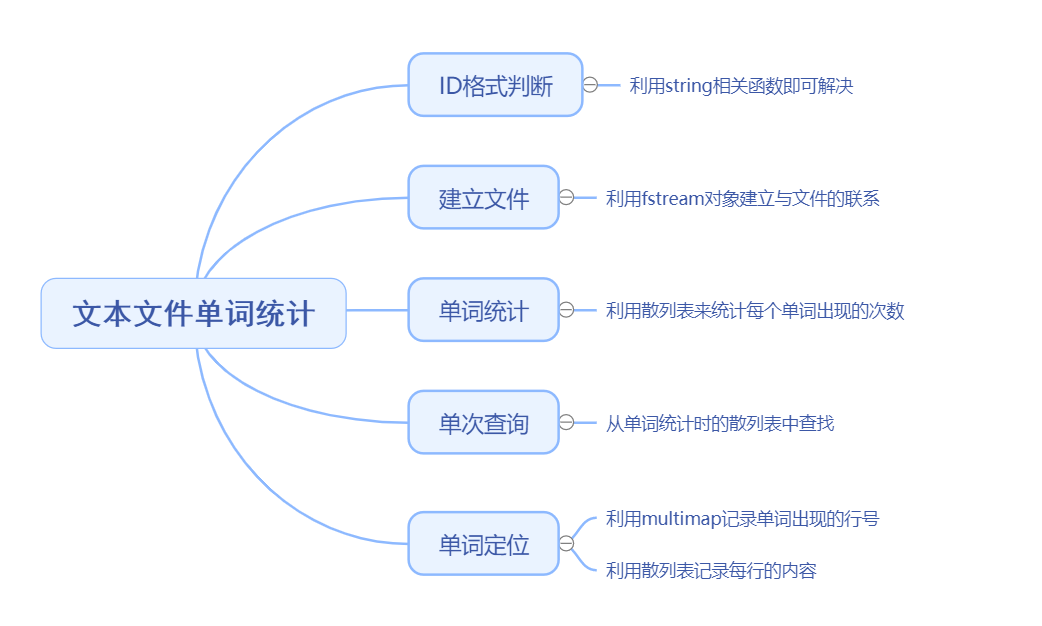接下来说说每个功能的实现：

### ID格式判断

这个很简单，直接上代码

//ID格式判断函数
bool idcheck(string str)
{
if(str.size() != 6) return false; //如果输入字符串长度不是6，直接返回false

for (int i = 0; i < str.size(); i++) //若长度是6，逐个字符遍历
{
if(i < 2)
{
if(!isupper(str[i])) return false; //如果前两位不是大写字母，返回false
}
else if(i >=2 && i < 6)
{
if(!isdigit(str[i])) return false; //如果后四位不是数字，返回false
}
}
return true; //如果上述条件都满足，返回true
}


因为题目要求ID长度为6，所以如果长度不是6直接返回，然后判断前两位是否是字母，后4位是否是数字。这个很简单，不再赘述。

### 建立文件

这里需要用到C++IO库中文件输入输出的知识，头文件fstream定义了三个类型来支持文件IO：ifstream从一个给定文件读取数据，ofstream向一个给定文件写入数据，以及fstream可以读写给定文件。本题中用到的是前两个类型ifstream和ofstream，顾名思义，i是input，代表输入，f是file，代表文件，用这个两个类型定义对象后，他们分别是默认从与之关联的文件中读和写的。ifstream是读，ofstream是写。

这里我们只需分别定义：

ifstream fin; //创建读文件的ifstream对象
ofstream fout; //创建写文件的ofstream对象


输出时的格式：

fstream的对象名 << "要输出的内容" << endl;


接下来我们要做的就是让ifstream对象和ofstream对象与相应的文件关联，关联的方式也很简单，直接用ifstream/ofstream对象的open()函数即可。函数参数为要关联的文件地址。我这里读入的文件名为Happiness.txt，路径在D盘编译环境的目录下。输出文件题目要求为soft.txt，我们直接用这个名字即可。open()函数要关联的对象如果不存在，会自动创建该文件。

还有一点要注意的是：访问文件一定要检查是否打开成功，如果没有打开成功就进行后续操作可能会产生意想不到的后果。然后就是对文件执行完相应的操作后，要用close函数关闭文件。

最后建立文件的功能集成到一个buildFile函数中，代码如下：

//建立文件
void bulidFile()
{
fin.open("D:\\Happiness.txt"); //这里的路径可以根据具体文件的路径来修改
if(!fin.is_open())
{
cout << "打开目标文件失败！" << endl;
return;
}

//创建我们将要输出数据的文件
//这里的路径可以根据具体文件的路径修改，如果目标文件不不存在将会创建该文件
fout.open("D:\\soft.txt");
if(!fout.is_open())
{
cout << "创建soft文件失败！" << endl;
return;
}

}


### 单词统计

单词统计的方法也不难想，要统计单词出现的频率，很直接的我们就想到了散列表（哈希表）因为一个string类型的数对应一个int类型的数用散列表可以很容易存储，C++中用unordered_map表示散列表。我们只需在读入单词的时候让键为该单词所对应的值+1即可。

我们定义一个散列表wordmap来存储单词出现的次数unordered_map<string, int> wordmap

unordered_map<string, int> wordmap; //记录单词出现次数的散列表
wordmap[word] ++;//这个单词的出现次数+1


wordmap[word] ++;的意思就是键为word的值自增，其中word是string类型的对象

一个重要的问题是：我们是按行读入的，那怎么样把单词拿出来单独判断呢？逐个字符判断的话非常不方便，而且还需要考虑空格问题。此时我们需要从string中读单词，我们可以使用C++IO库中的strIng流。

sstream头文件定义了三个类型文件来支持内存IO，这些类型可以向string写入数据，从string读取数据，就像string是一个IO流一样。

istringstream从string读取数据，ostringstream向string写入数据，而stringstream既可以从string读数据也可以向string写数据。

本题中只用istringstream对象便足以，我们需要创建一个istringstream对象并将其绑定到我们保存行的字符串，然后便可以从行中读取内容了。代码如下：

string line, word; //定义行和读入的单词
while(getline(fin, line)) //fin是我们之前创建的ifstream对象，用来从文件中读数据
{
istringstream oneline(line); //将istringstream对象online绑定到读入的行line
while(online >> word) //借助istringstream对象online从string对象line中读取单词
{
进行相应的单词处理、统计操作
}
}


解决了单词出现次数以及读入的问题，接下来要解决的是输出每个单词出现的次数。

因为单词是以key的形式存在散列表中的，输出不是很方便，这里我们用一个动态数组vector来输出。我们可以在读入单词的时候先判断一下该单词是否在散列表wordmap中出现过，如果没有出现过，就将其加入数组中（为什么要判断该单词是否出现过呢？因为我们输出单词清单时要求不能有重复，每个单词只能出现一次）。

动态数组的定义：vector<string> wordlist

wordlist.push_back(word)就是向动态数组中加入一个单词word。

当读完所有的文本之后我们遍历输出vector即可。

//遍历输出wordlist中的内容
for(auto c : wordlist)
{
fout << c << " 出现了 "<< wordmap[c] << "次" << endl;
}
/*auto是C++11标准中的编译器自动推断类型，意思就是编译器会自动把auto换成wordlist中元素的类型，当要遍历的对象类型名很长时，写auto就很方便，当然，这里的auto也可以换成string，因为wordlist中存的就是string类型的数，同理，如果vector存的是int,auto也可以换成int*/

//写成这样也可以
for(string c : wordlist)
{
fout << c << " 出现了 "<< wordmap[c] << "次" << endl;
}


单词的出现次数我们搞定了，接下来我们应该考虑一下读入单词时的处理，因为题目对单词的格式进行了一些限制：单词之间不止一个空格,且带引号的单词算单词，但数字不算单词，单词之间不止一个空格好说，按行读入的函数getline直接将一行读入，这个问题自动解决，我们要处理的就剩下带引号的单词这个问题。老规矩，也是把这个处理函数写成一个单词检查函数wordcheck()。先看下代码，结合代码理解。

#### 单词格式判断

//判断一个字符串是否是单词函数
bool wordCheck(string str)
{
for(int i = 0; i < str.size(); i ++ )
{
//第一种情况，带引号的单词
if(str == '\"') //对带引号的单词进行特判
{
for(int j = i + 1; j < str.size() - 1; j ++ ) //引号是单词开头的引号
{
if(!isalpha(str[j])) return false; //从引号下一个字符开始遍历
}
}
else //第二种情况，不带引号的单词
{
if(!isalpha(str[i])) return false;
}
}
return true;
}


wordcheck函数的返回值是布尔类型，如果传入的字符串符合单词的格式，返回true，否则返回false。

我们只需要判断传入字符串（单词）的第一位是否是引号，如果是引号，就从引号的下一位开始扫描，扫到最后一个引号的前一个位置为止，判断这些位置是否存在不是字母的情况。这里会用到字符函数，如下表。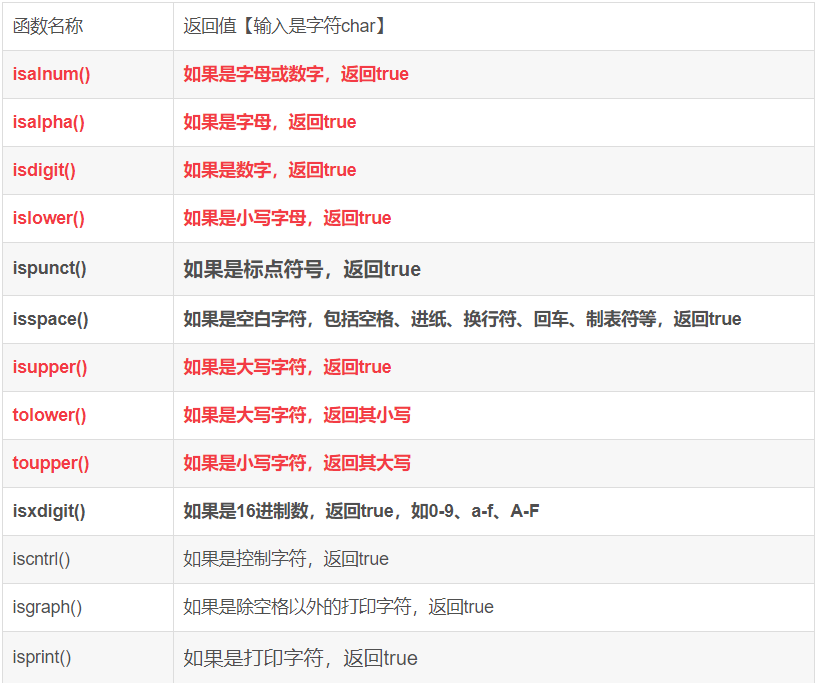到此为止，我们解决了单词的格式判断问题，还有一个要处理的地方，我们在输出单词出现次数的时候，因为要按小写abcd的形式排列，所以就不能出现大写字母，我们需要一个能把大写字母转换成小写字母的函数lowerword()。

#### 大写转小写函数

//大小写转换函数
string lowerword(string str)
{
string res;
for(int i = 0; i < str.size(); i ++)
{
str[i] = tolower(str[i]);
res += str[i];
}
return res;
}


这个没什么好说的，逐个字符扫描，用库函数tolower()即可，最后返回处理后的字符串。

然后，还有一个点，输出单词时要按abcd顺序输出，所以我们需要对vector数组中的元素按字典序进行排序，这里我们可以利用头文件<algorithm>中的sort()函数。

//将wordlist中的单词按字典序排序
sort(wordlist.begin(), wordlist.end());


呼~，到这里，单词统计功能就说完了。

### 单词查询

在我们做好单词统计的前期工作后，单词查询就变得非常简单了，直接从之前建立的散列表wordmap中查找输入的单词即可。

	cout << "请您输入待查询的单词：" << endl;
string word;
cin >> word;
if(wordmap.count(word)) //如果该单词在散列表中，进行相关操作
{
cout << "单词" << word << "共出现了" << wordmap[word] << "次；" << endl;
}


count()函数是unordered_map自带的函数，功能如下：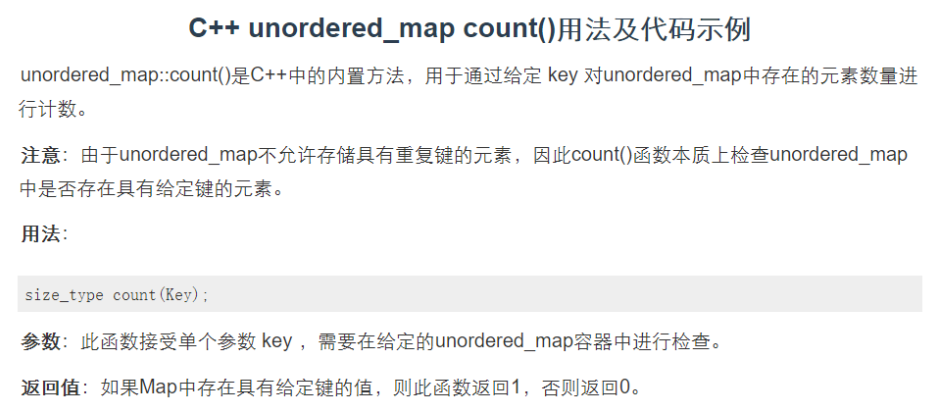说白了就是检查给定键是否在散列表中，如果在表中就返回1，否则返回0。

### 单词定位

题目要求输出查询单词的行号和具体位置，行号的话我们类比前面的单词出现次数，再定义一个散列表就行了，分别存单词和行号，然后再定义一个散列表来存行号和每行的内容。

但是，存单词和对应的行号用散列表真的合适吗？嘿嘿，既然我都这么问了，显然不合适，因为同一个单词可能在多行出现，此时，一个单词对应多个行号，即一个键对应多个值，此时散列表就不再适合存了，因为unordered_map要求一键对应一值，且键不允许重复。此时，我们应该用头文件<map>中的multimap来存单词和所在行号。multimap是允许一键对应多个值的，且multimap内部是有序的（其底层是红黑树，红黑树是一种弱化版的平衡二叉树）。

multimap<string, int> linemap; //记录单词对应的行号的散列表，用multimap是因为一个单词可能在多行出现
unordered_map<int, string> sentencemap; //记录每行的编号和内容
sentencemap.insert(make_pair(numline, line));//把每行编号和内容存到sentence中
linemap.insert(make_pair(res, numline)); //记录单词出现的行号，有可能出现一键多值的情况


单词存入上述两个容器中的过程在单词统计过程中实现，这里不再详细展开。

解决了存储同一单词对应多个行的问题，接下来的问题是我们如何访问一个键对应的多个值呢？也就是说我们如何从multimap中找到同一个单词对应的多个行呢？

解决这个问题需要用到迭代器的知识，大家只需要知道迭代器就是用来访问容器的一种工具，知道它的功能是什么就OK了，不要被它长长的定义格式吓到。

定义迭代器并赋值：

multimap<string, int>::iterator firstbucket; //这里用multimap是因为其有自动按键排序的功能
firstbucket = linemap.find(word); //find函数返回一个指向第一个具有键word元素的迭代器


firstbucket可以理解为一个链表（但它不是链表，指的是这种思想），它代表的是某个单词第一次出现的行号，继续向后遍历它，可以得到某个单词第二次、第三次···第N次出现的行号。

输出multimap中一键对应多值有两种方法：

//输出multimap中一键对应多值的方法一
multimap<string, int>::iterator firstbucket; //这里用multimap是因为其有自动按键排序的功能
firstbucket = linemap.find(word); //find函数返回一个指向第一个具有键word元素的迭代器
for(int k = 0; k != linemap.count(word); k ++, firstbucket ++)
{   //count函数求出某个键出现的次数
//firstbucket->second为单词出现的行数序号
cout << firstbucket->first << "——" << firstbucket->second << endl;
}

//输出multimap中一键对应多值的方法二
multimap<string, int>::iterator beg,end;
//equal_range函数返回一个迭代器的pair对象，first成员等价于lower_bound(key)，second成员等价于upper_bound(key)
/*  lower_bound(key)返回一个迭代器，指向键不小于k的第一个元素
upper_bound(key)返回一个迭代器，指向键不大于k的第一个元素*/
beg = linemap.equal_range(word).first;
end = linemap.equal_range(word).second;

for(firstbucket = beg; firstbucket != end; firstbucket ++)
{
cout << firstbucket->first << "——" << firstbucket->second << endl;
}



具体可以参考：C++multimap查找一个key对应的多个value

解决了找到同一单词对应的多个行的问题，接下来的问题就是如何定位单词在某一行的具体位置。

很直接的一个想法就是根据单词找到对应的行，然后扫描该行，如果该行存在一个词与输入的单词相同，记录下标，然后输出即可。但是，如果该行存在多个词与输入的单词相同会发生什么呢？

int index = 0,location = 0;
while(读入单词)
{
index ++;
if(word == 行中的某个单词)
{
location = index;
}
}
cout << "单词在该行的第" << location << "个位置" << endl;


按照上面的代码，index会被更新多次（这个次数为该单词在同一行出现的次数），前面index的值会被后面index的值覆盖掉，所以最后输出的index是该单词在该行最后一次出现的位置。

举个例子：

测试文本的第27行中单词“and”出现了2次，第一次在第2个位置，第二次在第7个位置

life and suggests how these emotions and sense of meaning reinforce one another.

如果我们直接用上面的思路，最后输出的index应该都是7。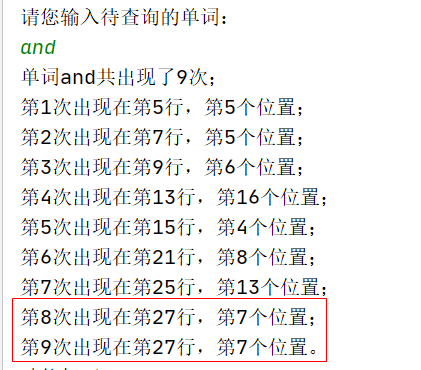解决这个问题的办法是设置一个标志数组st[]，每当在某行找到一个与待查询单词匹配的单词时，就将其标志为true，每次访问单词时检查该单词是否被访问过，如果访问过就跳过该单词，继续寻找下一个。如果没有访问过就可以输出Index下标。

具体代码如下：

			while(is >> str) //从单词出现的行逐个读入单词，is是istringstream对象
{
index ++;
if(str == word)
{
if(!st[index]) //如果当前单词之前没有被访问过，更新下标以及访问标记
{
location = index;
st[index] = true;
break;
//如果匹配到了，就跳出循环，不然程序还会向后扫描，这样就把之前的第一个位置的下标覆盖掉了
//结果输出的都是最后出现位置的下标
}
else continue; //该单词之前被访问过，跳过该单词，继续扫描下一个。
}

}


增加标志数组后，输出结果就正确了：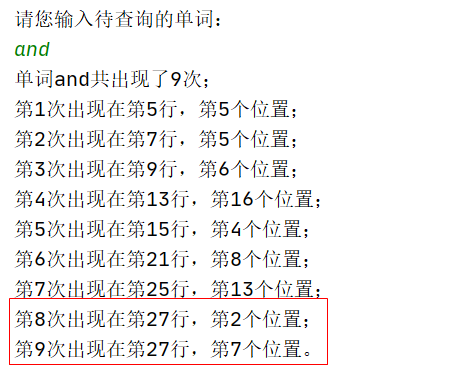至此，所有功能就已全部实现了。

下面是完整的源程序代码：

#include <iostream>
#include <cstring>
#include <algorithm>
#include <fstream>
#include <sstream>
#include <map>
#include <unordered_map>
#include <vector>

using namespace std;

const int N = 1000; //设置这里主要是设置标志数组的大小，一般文本单词数在1000以内，故设1000

int cnt = 0; //单词总数
int numline = 0; //行号
ifstream fin; //创建读文件的ifstream对象
ofstream fout; //创建写文件的ofstream对象
unordered_map<string, int> wordmap; //记录单词出现次数的散列表
multimap<string, int> linemap; //记录单词对应的行号的散列表，用multimap是因为一个单词可能在多行出现
unordered_map<int, string> sentencemap; //每行的编号和内容
vector<string> wordlist; //存单词列表（不重复）
vector<string> linelist; //每次读入一行后，把读入的行加入到linelist中去，最后linelist存的其实是整篇文章

//函数声明
bool idcheck(string str); //ID格式判断函数
string lowerword(string str); //将大写转换为小写函数
bool wordcheck(string str); //判断一个字符串是否是单词函数
void buildFile(); //文件建立函数
void wordCount(); //单词统计函数
void wordLocal(); //单词查询及定位函数

//ID格式判断函数
bool idcheck(string str)
{
if(str.size() != 6) return false; //如果输入字符串长度不是6，直接返回false

for (int i = 0; i < str.size(); i++) //若长度是6，逐个字符遍历
{
if(i < 2)
{
if(!isupper(str[i])) return false; //如果前两位不是大写字母，返回false
}
else if(i >=2 && i < 6)
{
if(!isdigit(str[i])) return false; //如果后四位不是数字，返回false
}
}
return true; //如果上述条件都满足，返回true
}

//大小写转换函数
string lowerword(string str)
{
string res;
for(int i = 0; i < str.size(); i ++)
{
str[i] = tolower(str[i]);
res += str[i];
}
return res;
}

//判断一个字符串是否是单词函数
bool wordCheck(string str)
{
for(int i = 0; i < str.size(); i ++ )
{
//第一种情况，带引号的单词
if(str == '\"') //对带引号的单词进行特判
{
for(int j = i + 1; j < str.size() - 1; j ++ ) //引号是单词开头的引号
{
if(!isalpha(str[j])) return false; //从引号下一个字符开始遍历
}
}
else //第二种情况，不带引号的单词
{
if(!isalpha(str[i])) return false;
}
}
return true;
}

//建立文件
void bulidFile()
{
fin.open("D:\\Happiness.txt"); //这里的路径可以根据具体文件的路径来修改
if(!fin.is_open())
{
cout << "打开目标文件失败！" << endl;
return;
}

//创建我们将要输出数据的文件
//这里的路径可以根据具体文件的路径修改，如果目标文件不不存在将会创建该文件
fout.open("D:\\soft.txt");
if(!fout.is_open())
{
cout << "创建soft文件失败！" << endl;
return;
}

cout << "已建立（打开）单词文件，您现在可以使用2,3功能！" << endl;
}

//单词统计
void wordCount()
{
string line; //定义行
string word;//读取文件中的单词

while(getline(fin, line)) //按行读取,存到字符串line中
{
++ numline; //行号加一
linelist.push_back(line); //把这一行存到linelist中

sentencemap.insert(make_pair(numline, line));//把每行编号和内容存到sentence中

istringstream oneline(line); //创建一个istringstream对象与line绑定，为了从字符串对象line中读单词
while(oneline >> word)
{
if(wordCheck(word)) //如果是符合要求的单词
{
string res = lowerword(word); //把单词转换成小写
cnt ++; //文档单词总数加1
if(!wordmap.count(res)) //如果这个单词之前没有出现过
{//就把它加入到vector数组中，我们要保证vector中的单词不重复出现
wordlist.push_back(res);
}
wordmap[res] ++;//这个单词的出现次数+1
linemap.insert(make_pair(res, numline)); //记录单词出现的行号，有可能出现一键多值的情况
}
}
}

fout << "文档中单词总数:" << cnt << endl;
fout << "各个单词出现的频次：" << endl;

//将wordlist中的单词按字典序排序
sort(wordlist.begin(), wordlist.end());

//遍历输出wordlist中的内容
for(auto c : wordlist)
{
fout << c << " 出现了 "<< wordmap[c] << "次" << endl;
}

fin.close(); //读取完了文件，需要关闭
fout.close();

cout << "单词统计完成，请到soft.txt文件查看结果！" << endl;
}

//单词查询及定位
void wordLocal()
{
cout << "请您输入待查询的单词：" << endl;
string word;
cin >> word;
if(wordmap.count(word)) //如果该单词在散列表中，进行相关操作
{
cout << "单词" << word << "共出现了" << wordmap[word] << "次；" << endl;
int num = wordmap[word];
int location = 0;

multimap<string, int>::iterator firstbucket; //这里用multimap是因为其有自动按键排序的功能
firstbucket = linemap.find(word); //find函数返回一个指向第一个具有键word元素的迭代器

int numtimes = 1; //单词出现次数
bool st[N]; //定义标志数组，用来标记下标为index的单词是否被访问过
for(int i = 0; i < N; i ++ ) st[i] = false; //初始化标志数组

//输出multimap中一键对应多值的方法一
for(int k = 0; k != linemap.count(word); k ++, firstbucket ++)
{   //count函数求出某个键出现的次数
//firstbucket->second为单词出现的行数序号
//location = sentencemap[firstbucket->second].find(word);
istringstream is(sentencemap[firstbucket->second]); //将单词出现的行绑定到string流
string str; //每次读入一个单词
int index = 0; //定义下标

while(is >> str) //从单词出现的行逐个读入单词
{
index ++;
if(str == word)
{
//cout << "当前单词序号：" << index << endl;
if(!st[index]) //如果当前单词之前没有被访问过，更新下标以及访问标记
{
location = index;
st[index] = true;
break;
//如果匹配到了，就跳出循环，不然程序还会向后扫描，这样就把之前的第一个位置的下标覆盖掉了
//结果输出的都是最后出现位置的下标
}
else continue; //该单词之前被访问过，跳过该单词，继续扫描下一个。
}

}

cout << "第" << numtimes << "次出现在第" << firstbucket->second << "行，第" << location << "个位置";
if(numtimes == num) cout << "。" << endl;
else cout << "；" << endl;
numtimes ++;
}
//每当一行扫描完成后，复原标志数组，不然会影响后面扫描其他行的结果
for(int i = 0; i < N; i ++ ) st[i] = false;

//输出multimap中一键对应多值的方法二
//        multimap<string, int>::iterator beg,end;
//        //equal_range函数返回一个迭代器的pair对象，first成员等价于lower_bound(key)，second成员等价于upper_bound(key)
//        beg = linemap.equal_range(word).first;
//        end = linemap.equal_range(word).second;
//
//        int numtimes = 1;
//        for(firstbucket = beg; firstbucket != end; firstbucket ++)
//        {
//            cout << "第" << numtimes << "次出现在第" << firstbucket->second << "行，第" << location << "个位置" << endl;
//            numtimes ++;
//        }
}
else //如果该单词不在散列表中，输出查无此词。
{
cout << "查无此词！" << endl;
}
}

int main()
{
bool flag = false; //定义一个flag表示是否已建立文件
while (true)
{
cout << "请输入用户ID：" << endl;
string str;
cin >> str;
if(!idcheck(str))
{
cout << "ID格式有误，请重新输入！" << endl;
}
else
{
while(true)
{
cout << "功能如下：" << endl;
cout << "(1)建立文件" << endl;
cout << "(2)单词统计" << endl;
cout << "(3)单词查询及定位" << endl;
cout << "(4)退出" << endl;

cout << "请输入您的选择：" << endl;
string str1;
cin >> str1;
int num1 = 0;
for(int i = 0; i < str1.size(); i++)
{
int x = str1[i] - '0';
num1 = num1 * 10 + x;
}
if(num1 > 4 || num1 < 1) cout << "只能输入1-4之间的数，请重新输入!" << endl;
else if(num1 == 1)
{
flag = true;
bulidFile();
}
else if(num1 == 2)
{
if(!flag) cout << "您还没有建立文件，请先使用1功能建立文件！" << endl;
else
{
wordCount();
}
}
else if(num1 == 3)
{
if(!flag) cout << "您还没有建立文件，请先使用1功能建立文件！" << endl;
else
{
wordLocal();
}
}
else if(num1 == 4)
{
cout << "感谢使用！" << endl;
return 0;
}
}
}
}
return 0;
}


下面附上测试文件Happiness.txt的原文

What Is Happiness

Most of us probably don’t believe we need a formal definition of happiness; we know it

when we feel it, and we often use the term to describe a range of positive emotions,

including joy, pride, contentment, and gratitude.

But to understand the causes and effects of happiness, researchers first need to define

it. Many of them use the term interchangeably with “subjective well-being,” which they

measure by simply asking people to report how satisfied they feel with their own lives and

how much positive and negative emotion they’re experiencing. In her 2007 book The How

of Happiness, positive psychology researcher Sonja Lyubomirsky elaborates, describing

happiness as “the experience of joy, contentment, or positive well being, combined with a

sense that one’s life is good, meaningful, and worthwhile.”

That definition resonates with us here at Greater Good: It captures the fleeting positive

emotions that come with happiness, along with a deeper sense of meaning and purpose in

life and suggests how these emotions and sense of meaning reinforce one another.



程序运行结果：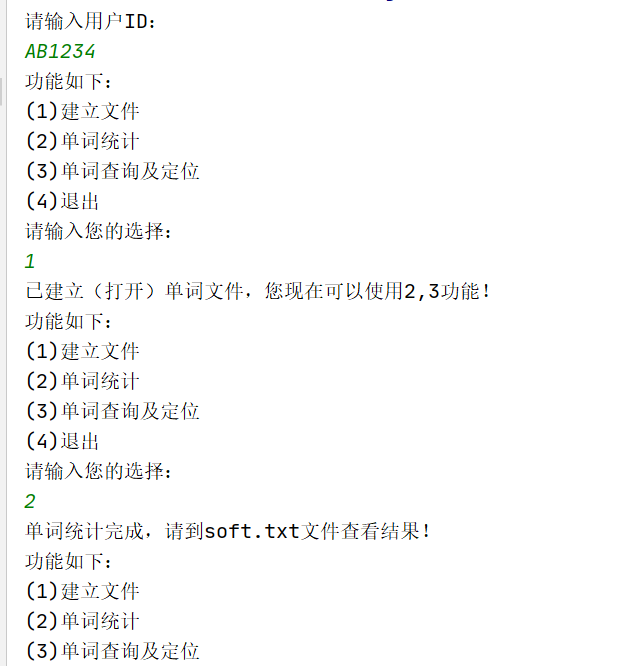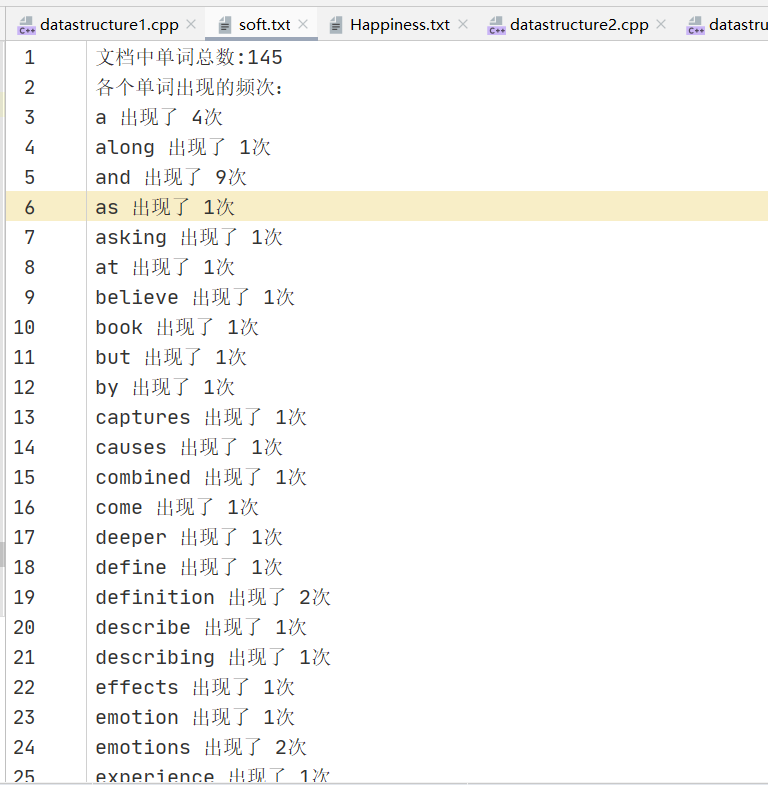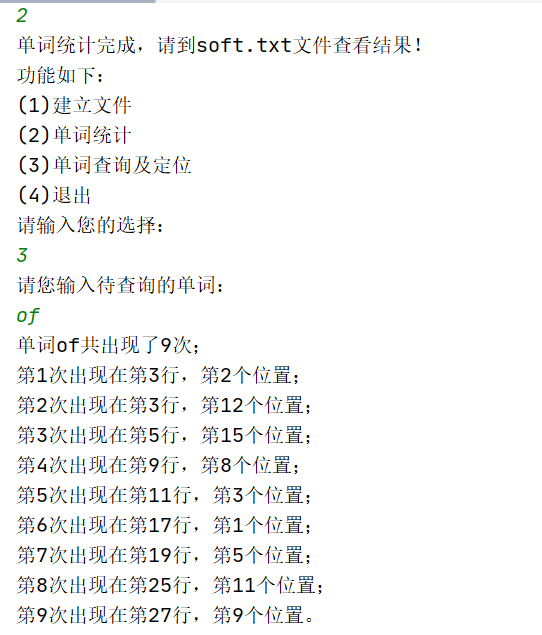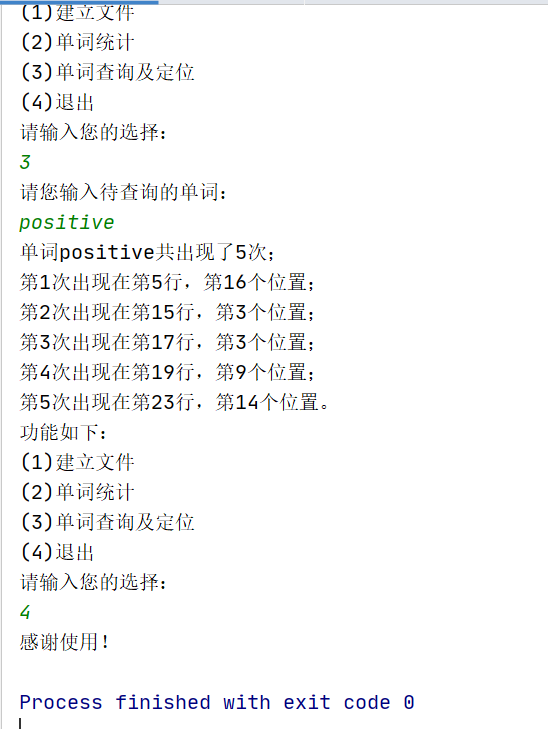原创不易，如果本文对你有帮助，可以点个赞支持一下~

展开全文c++
• 主要介绍了python统计文本文件单词数量的方法,涉及Python针对文本文件及字符串的相关操作技巧,需要的朋友可以参考下
• 主要介绍了Python统计文本文件中英文单词出现个数的方法,结合实例形式总结分析了Python针对文本文件的读取,以及统计文本文件中英文单词个数的4种常用操作技巧,需要的朋友可以参考下
• 主要为大家详细介绍了C语言实现英文文本词频统计，文中示例代码介绍的非常详细，具有一定的参考价值，感兴趣的小伙伴们可以参考一下
• 统计给定单词文本文件中出现的总次数；检索输出某个单词出现在文本中的行号、在该行中出现的次数以及位置。 该设计要求可分为三个部分实现：其一，建立文本文件，文件名由用户用键盘输入；其二，给定单词的计数，...
•c语言
• 编写一个文本文件单词统计的程序，包括建立文件、单词统计、单词查询、单词定位的功能。 1.2 【基本要求】 程序应先询问用户的ID号(ID号包括两个大写字母和4 位数字)，例如: 请输入用户 ID 号 :AB1234 程序应对输入...
• 本文实例为大家分享了python统计文本单词出现频率的具体代码，供大家参考，具体内容如下 #coding=utf-8 import os from collections import Counter sumsdata=[] for fname in os.listdir(os.getcwd()): if os....
• 编写一个程序，分析一个文本文件（英文...文本文件为D:\test.txt ，输出该文本文件中含有的单词总数，分析一个文本文件（英文文章）中 各个词出现的频率，输出该文本文件中所有单词的列表，并与出现次数一起打印出来
• 可以用简单的图形界面显示文本所有英文单词的数目，并可以查询固定单词的个数
• 本文通过实例代码给大家介绍的C语言统计一篇英文短文中单词的个数，代码简单易懂，非常不错，具有参考借鉴价值，需要的朋友参考下吧
• 文本文件单词统计排序(报告加源码),C++6.0下验证通过,无误,赚点下载积分糊口....
• 测试文件统计在C：\ temp创建一个名为temp的文件夹创建要计算的文本的文本文件C++
• 已结贴√问题点数：40回复次数：20 统计一个文本文件中的单词个数和汉字个数统计一个文本文件中的单词个数和汉字个数我在word里面输入了一段中文，一段英文 看它统计的数据(比如单词99 ，汉字99)然后用一下程序实现...
• 文章目录一、文本文档信息存储展示 一、文本文档信息存储展示
• 问题描述：编写一个文本文件单词统计的程序，包括建立文件、单词统计、单词查询、单词定位的功能。 基本要求： 程序应先询问用户的 ID号(ID 号包括两个大写字母和4 位数字)，例如: 请输入用户 ID 号:AB1234 程序应对...java
• 统计一行文本单词个数:输入一行字符,统计其中单词的个数.个单词之间用空格分隔，空格数可以是多个，代码部分： #includeint main(){int count=0;char temp;bool letter;letter=0;//letter=0为在单词里(单词没......